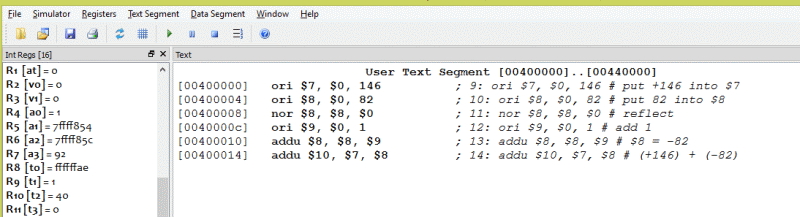146 - 82 = 64       in hex = 0x40

# Sign ExtensionA run of the program produces the expected result.

So that one could be added to register `\$8`, the one was first loaded into another register. It would be nice if there were an "add one" instruction. Many processors have such an instruction.

MIPS has an "add immediate unsigned" instruction: `addiu d,s,const`. It can be used to add one to register `\$8` like this:

`addiu \$8,\$8,1`

The immediate operand of this instruction is 16 bits (as are all MIPS immediate operands). However, when extended to a 32-bit operand by the ALU it is sign extended: The value of the left-most bit of the immediate operand (bit 15) is copied to all bits to the left (into the high-order bits). So if the 16-bit immediate operand is a 16-bit two's complement negative integer, the 32-bit ALU operand is a 32-bit version of the same negative integer. The left-most bit of a two's complement integer is sometimes called the "sign bit".

### QUESTION 9:

Here is a 16-bit two's complement negative one:

`1111 1111 1111 1111`

Sign-extend it to 32 bits:

Here is a 16-bit two's complement negative one written in hex:

`FFFF`

Write a 32-bit negative one in hex: